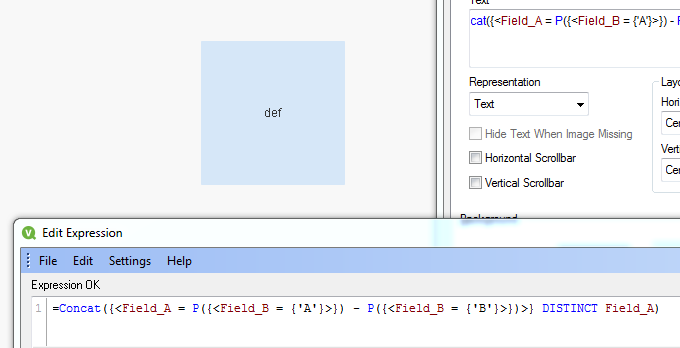# QlikView App Development

Discussion Board for collaboration related to QlikView App Development.

Announcements
BARC’s The BI Survey 19 makes it official. BI users love Qlik. GET REPORT
HighlightedNot applicable

## exclusion in set analysis

Hi

I have a table which looks like this

Field_AField_B

a

A
bA
cA
dA
eA
fA
aB
bB
cB

I want the list of Field_A items which are A but not B (which would be here "def")

I was thinking of doing something like :

=concat( {<Field_B={"A"} > -   <Field_B={"B"}> }   DISTINCT Field_A)

But it doesn't seem to work :

it returns "abcdef" instead of "def"

Could you help me out ?

Thanks !

Tags (2)
1 Solution

Accepted SolutionsMVP

## Re: exclusion in set analysis

May be like this:

=Concat({<Field_A = P({<Field_B = {'A'}>}) - P({<Field_B = {'B'}>})>} DISTINCT Field_A)11 RepliesMVP

## Re: exclusion in set analysis

May be like this:

=Concat({<Field_A = P({<Field_B = {'A'}>}) - P({<Field_B = {'B'}>})>} DISTINCT Field_A)Not applicable

## Re: exclusion in set analysis

That's it thanks a lot for your help !MVP

## Re: exclusion in set analysis

No problem at allEsteemed Contributor III

## Re: exclusion in set analysis

Try this:

Table1:

Field_A, Field_B

a,  A

b, A

c, A

d, A

e, A

f, A

a, B

b, B

c, B

];

JOIN(Table1)

Field_B

Resident Table1

WHere Field_B <> 'B';MVP

## Re: exclusion in set analysis

maybe

=Concat({\$ <Field_B={A},Field_A={"=count(distinct Field_B)=1"}>} Field_A)Not applicable

## Re: exclusion in set analysis

Sunny T,

Let's say, I have another Field, Filed_C, and i want to disregard any selection made in that field.

I tried both this formulas, but it doen't to what expected.

Any idea ?

=Concat({<Field_A = P({<Field_B = {'A'} , Field_C= >}) - P({<Field_B = {'B'}> , Field_C= })>} DISTINCT Field_A)

=Concat({< Field_C=, Field_A = P({<Field_B = {'A'} >}) - P({<Field_B = {'B'}>  })>} DISTINCT Field_A)MVP

## Re: exclusion in set analysis

Try this:

=Concat({<Field_A = P({<Field_B = {'A'}>}) - P({<Field_B = {'B'}>}), Field_C=>} DISTINCT Field_A)Not applicable

## Re: exclusion in set analysis

Hey

Unfortunately it doesn't work.

Your last formula has the same result as your first formula when I do a selection on Field_CMVP